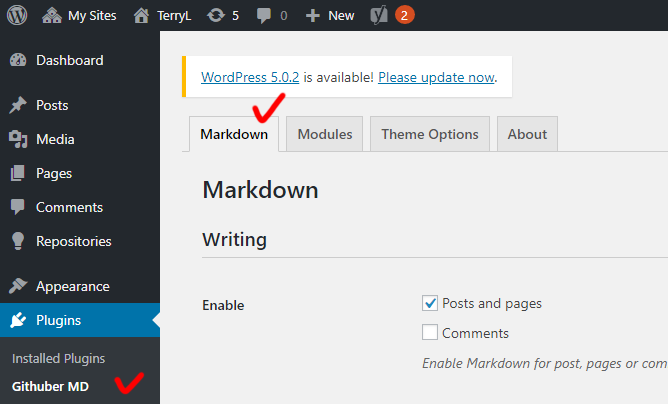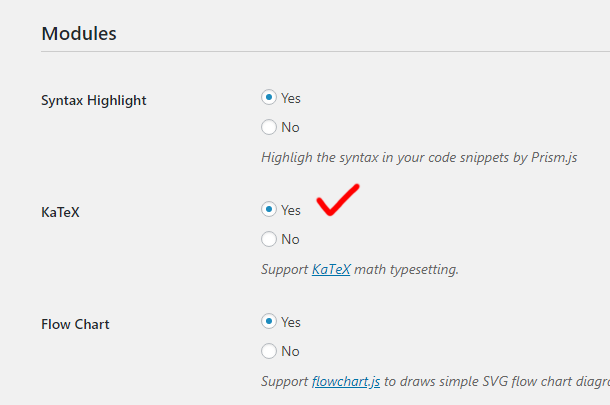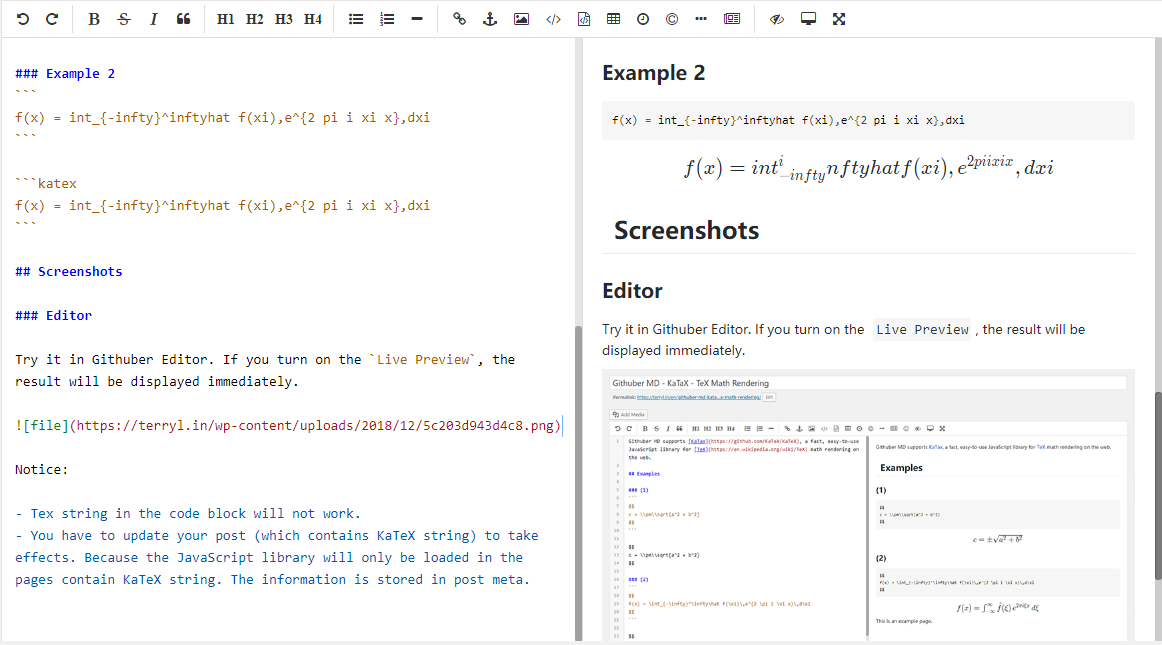Githuber MD supports KaTax, a fast, easy-to-use JavaScript library for TeX math rendering on the web.

## Steps

Follow these steps to enable KaTax module.(1) Click “Githuber MD” and in the “Markdown” settings page.(2) click “Yes” for option “KaTex”.

## Examples

### Example 1

c = \pm\sqrt{a^2 + b^2}
c = \pm\sqrt{a^2 + b^2}

### Example 2

f(x) = int_{-infty}^inftyhat f(xi),e^{2 pi i xi x},dxi
f(x) = int_{-infty}^inftyhat f(xi),e^{2 pi i xi x},dxi

## Screenshots

### Editor

Try it in Githuber Editor. If you turn on the Live Preview, the result will be displayed immediately.Notice:

• You have to update your post (which contains KaTeX string) to take effects. Because the JavaScript library will only be loaded in the pages contain KaTeX string. The information is stored in post meta.

### AuthorHi, thank you for your plugin. In the live previw, the KaTex works well, but when the post has been published or updated, it can not work. For example, it shows “c = \pm\sqrt{a^2 + b^2}”in the post. The version of wordpress is 5.2You have to specify the type code on the markdown code block to make it work, for example:
katex c = \pm\sqrt{a^2 + b^2}Paragraph with $$sin(x)$$ desn’t seem to work in online editor :(. Is there way to put inline math to the post? KaTeX support inline math. Thanks 🙂$$f(x) = \int_{-\infty}^\infty\hat f(\xi)\,e^{2 \pi i \xi x}\,d\xi$$